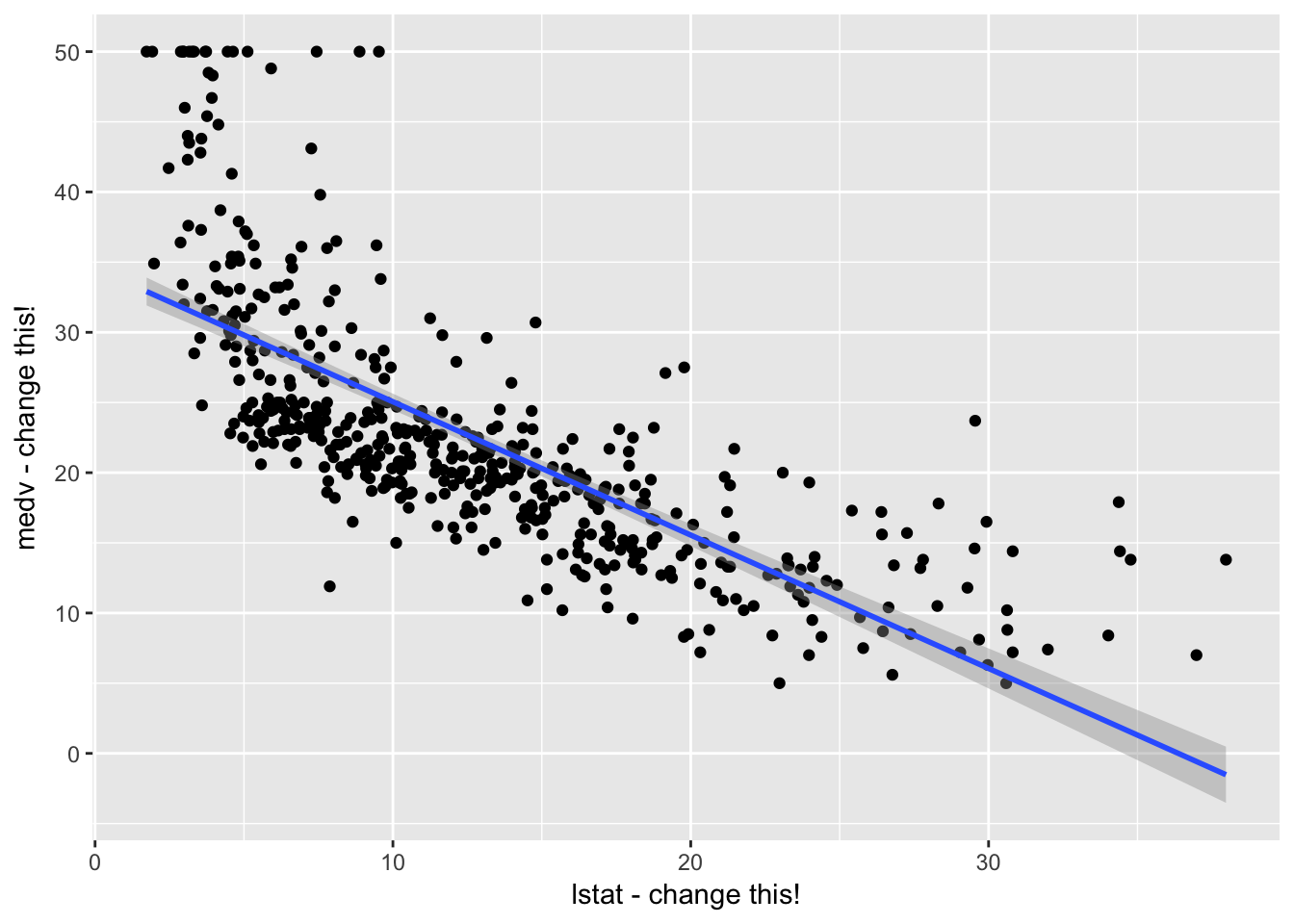### 1. Changing the author field and file name.

##### (b) Rename this file to “lab01_YourHameHere.Rmd”, where YourNameHere is changed to your own name.

Just like every other programming language you may be familiar with, R’s capabilities can be greatly extended by installing additional “packages” and “libraries”.

To install a package, use the install.packages() command. You’ll want to run the following commands to get the necessary packages for today’s lab:

install.packages("ggplot2")
install.packages("MASS")
install.packages("ISLR")
install.packages("knitr")

You only need to install packages once. Once they’re installed, you may use them by loading the libraries using the library() command. For today’s lab, you’ll want to run the following code

library(ggplot2) # graphics library
library(MASS)    # contains data sets, including Boston
library(ISLR)    # contains code and data from the textbook
library(knitr)   # contains kable() function

options(scipen = 4)  # Suppresses scientific notation

### 3. Simple Linear Regression with the Boston Housing data.

This portion of the lab gets you to carry out the Lab in §3.6 of ISLR (Pages 109 - 118). You will want to have the textbook Lab open in front you as you go through these exercises. The ISLR Lab provides much more context and explanation for what you’re doing.

Please run all of the code indicated in §3.6 of ISLR, even if I don’t explicitly ask you to do so in this document.

Note: You may want to use the View(Boston) command instead of fix(Boston).

##### (a) Use the dim() command to figure out the number of rows and columns in the Boston housing data
# Edit me
##### (b) Use the nrow() and ncol() commands to figure out the number of rows and columns in the Boston housing data.
# Edit me
##### (c) Use the names() command to see which variables exist in the data. Which of these variables is our response variable? What does this response variable refer to? How many input variables do we have?
# Edit me
##### (d) Use the lm() function to a fit linear regression of medv on lstat. Save the output of your linear regression in a variable called lm.fit.
# Edit me
##### (e) Use the summary() command on your lm.fit object to get a print-out of your regression results
# Edit me
##### (f) Uncomment the line below to get a ‘nice’ printout of the coefficients table
# kable(coef(summary(lm.fit)), digits = c(4, 5, 2, 4))
##### (g) Call names() on lm.fit to explore what values this linear model object contains.
# Edit me
##### (h) Use the coef() function to get the estimated coefficients. What is the estimated Intercept? What is the coefficient of lstat in the model? Interpret this coefficient.
# Edit me
##### (i) Here’s a ggplot command that overlays a linear regression line on a scatterplot of mdev vs. lstat. Edit the xlab and ylab arguments to produce more meaningful axis labels. Does the linear model appear to fit the data well? Explain.
qplot(data = Boston, x = lstat, y = medv,
xlab = "lstat - change this!", ylab = "medv - change this!") + stat_smooth(method = "lm")##### (i) Follow the ISLR examples for getting confidence intervals and prediction intervals for the regression data.
# Fill in later

### 4. Multiple Linear Regression with the Boston Housing data

##### (b) Following the example in part 3(i) of this lab, use the qplot() command to construct a scatterplot of medv veruses age. Make sure to specify meaningful x and y axis names. Overlay a linear regression line. Does a linear relationship appear to hold between the two variables?
# Edit me
##### (c) Use the lm() command to a fit a linear regression of medv on lstat and age. Save your regression model in a variable called lm.fit.
# Edit me
##### (d) What is the coefficient of age in your model? Interpret this coefficient.
# Edit me
##### (e) Use medv ~ . syntax to fit a model regressing medv on all the other variables. Use the summary() and kable() functions to produce a coefficients table in nice formatting.
# Edit me
##### (f) Think about what the variables in the data set mean. Do the signs of all of the coefficient estimates make sense? Are there any that do not? For the ones that do not, are the coefficients statistically significant (do they have p-value < 0.05)?
# Edit me

### 5. Non-linear transformations of the predictors

##### (a) Perform a regression of medv onto a quadratic polynomial of lstat by using the formula medv ~ lstat + I(lstat^2). Use the summary() function to display the estimated coefficients. Is the coefficient of the squared term statistically significant?
# Edit me
##### (b) Try using the formula medv ~ lstat + lstat^2 instead. What happens?
# Edit me
##### (c) Use the formula medv ~ poly(lstat, 2). Compare your results to part (a).
# Edit me

### 6. ggplot visualizations

ggplot’s stat_smooth command allows us to visualize simple regression models in a really easy way. This set of problems helps you get accustomed to specifying polynomial and step function formulas for the purpose of visualization.

For this problem, please refer to the code posted here: Week 1 R code

##### (a) Use ggplot graphics to construct a scatterplot of medv vs lstat, overlaying a 2nd degree polynomial. Does this appear to be a good model of the data? Construct plots with higher degree polynomial fits. Do any of them appear to describe the data particularly well?
# Edit me
##### (c) Repeat part (a), this time using ptratio as the x-axis variable, and medv still as the y-axis variable.
# Edit me
##### (d) Repeat part (b), this time with ptratio instead of lstat.
# Edit me